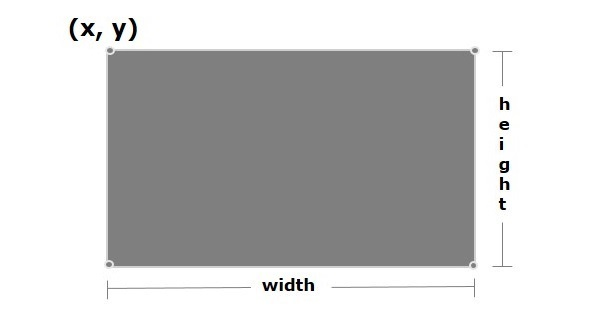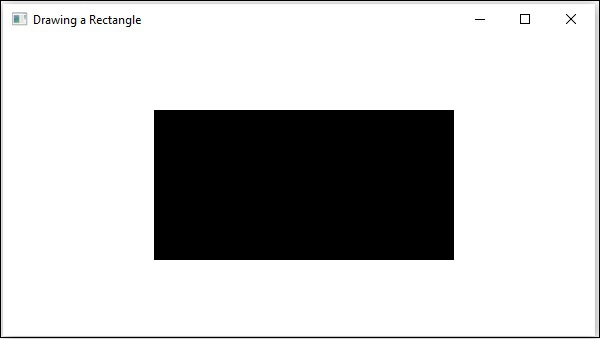# JavaFX - 2D Shapes Rectangle

In general, a rectangle is a four-sided polygon that has two pairs of parallel and concurrent sides with all interior angles as right angles.

It is described by two parameters namely −

• height − The vertical length of the rectangle is known as height.

• width − The horizontal length of the rectangle is known as width.In JavaFX, a Rectangle is represented by a class named Rectangle. This class belongs to the package javafx.scene.shape.

By instantiating this class, you can create a Rectangle node in JavaFX.

This class has 4 properties of the double datatype namely −

• X − The x coordinate of the start point (upper left) of the rectangle.

• Y − The y coordinate of the start point (upper left) of the rectangle.

• Width − The width of the rectangle.

• height − The height of the rectangle.

To draw a rectangle, you need to pass values to these properties, either by passing them to the constructor of this class, in the same order, at the time of instantiation, as shown below −

```Rectangle rectangle = new Rectangle(x, y, width, height);
```

Or, by using their respective setter methods as shown in the following code block −

```setX(value);
setY(value);
setWidth(value);
setHeight(value);
```

## Steps to Draw a Rectangle

You need to follow the steps given below to draw a rectangle in JavaFX.

### Step 1: Creating a Class

Create a Java class and inherit the Application class of the package javafx.application and implement the start() method of this class as shown below.

```public class ClassName extends Application {
@Override
public void start(Stage primaryStage) throws Exception {
}
}
```

### Step 2: Creating a Rectangle

You can create a rectangle in JavaFX by instantiating the class named Rectangle which belongs to a package javafx.scene.shape, instantiate this class as follows.

```//Creating a rectangle object
Rectangle rectangle = new Rectangle();
```

### Step 3: Setting Properties to the Rectangle

Specify the x, y coordinates of the starting point (upper left), height and the width of the rectangle, that is needed to be drawn. You can do this by setting the properties x, y, height and width, using their respective setter methods as shown in the following code block.

```line.setStartX(100.0);
line.setStartY(150.0);
line.setEndX(500.0);
line.setEndY(150.0);
```

### Step 4: Creating a Group Object

In the start() method, create a group object by instantiating the class named Group, which belongs to the package javafx.scene.

Pass the Rectangle (node) object, created in the previous step, as a parameter to the constructor of the Group class, in order to add it to the group as follows −

```Group root = new Group(rectangle);
```

### Step 5: Creating a Scene Object

Create a Scene by instantiating the class named Scene that belongs to the package javafx.scene. To this class, pass the Group object (root), created in the previous step.

In addition to the root object, you can also pass two double parameters representing height and width of the screen along with the object of the Group class as follows.

```Scene scene = new Scene(group ,600, 300)
```

### Step 6: Setting the Title of the Stage

You can set the title to the stage using the setTitle() method of the Stage class. The primaryStage is a Stage object, which is passed to the start method of the scene class, as a parameter.

Using the primaryStage object, set the title of the scene as Sample Application as follows.

```primaryStage.setTitle("Sample Application");
```

### Step 7: Adding Scene to the Stage

You can add a Scene object to the stage using the method setScene() of the class named Stage. Add the Scene object prepared in the previous steps using the method shown below.

```primaryStage.setScene(scene)
```

### Step 8: Displaying the Contents of the Stage

Display the contents of the scene using the method named show() of the Stage class as follows.

```primaryStage.show()
```

### Step 9: Launching the Application

Launch the JavaFX application by calling the static method launch() of the Application class from the main method as follows.

```public static void main(String args[]){
launch(args);
}
```

### Example

Following is the program which generates a rectangle JavaFX. Save this code in a file with the name RectangleExample.java.

```import javafx.application.Application;
import javafx.scene.Group;
import javafx.scene.Scene;
import javafx.stage.Stage;
import javafx.scene.shape.Rectangle;

public class RectangleExample extends Application {
@Override
public void start(Stage stage) {
//Drawing a Rectangle
Rectangle rectangle = new Rectangle();

//Setting the properties of the rectangle
rectangle.setX(150.0f);
rectangle.setY(75.0f);
rectangle.setWidth(300.0f);
rectangle.setHeight(150.0f);

//Creating a Group object
Group root = new Group(rectangle);

//Creating a scene object
Scene scene = new Scene(root, 600, 300);

//Setting title to the Stage
stage.setTitle("Drawing a Rectangle");

stage.setScene(scene);

//Displaying the contents of the stage
stage.show();
}
public static void main(String args[]){
launch(args);
}
}
```

Compile and execute the saved java file from the command prompt using the following commands.

```javac RectangleExample.java
java RectangleExample
```

On executing, the above program generates a JavaFX window displaying a rectangle as shown in the following screenshot.javafx_2d_shapes.htm